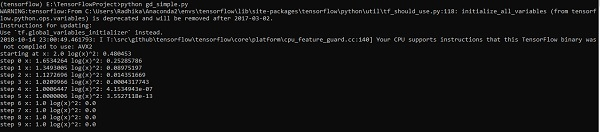# TensorFlow - Gradient Descent Optimization

Gradient descent optimization is considered to be an important concept in data science.

Consider the steps shown below to understand the implementation of gradient descent optimization −

## Step 1

Include necessary modules and declaration of x and y variables through which we are going to define the gradient descent optimization.

import tensorflow as tf

x = tf.Variable(2, name = 'x', dtype = tf.float32)
log_x = tf.log(x)
log_x_squared = tf.square(log_x)

train = optimizer.minimize(log_x_squared)


## Step 2

Initialize the necessary variables and call the optimizers for defining and calling it with respective function.

init = tf.initialize_all_variables()

def optimize():
with tf.Session() as session:
session.run(init)
print("starting at", "x:", session.run(x), "log(x)^2:", session.run(log_x_squared))

for step in range(10):
session.run(train)
print("step", step, "x:", session.run(x), "log(x)^2:", session.run(log_x_squared))
optimize()


The above line of code generates an output as shown in the screenshot below −We can see that the necessary epochs and iterations are calculated as shown in the output.